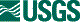## ISIS 2ISIS Application Documentation

# ratio

Printer Friendly View | TOC | Home

Divide two cubes

 Overview Parameters Example 1 Example 2

## Description

This program divides two cubes. It operates in one of two manners: 1) the denominator cube must have the same number of bands as the numerator cube or 2) the denominator cube must have exactly one band. In the former case, corresponding bands are divided, for example, band one is divided by band one, band two by band two, and so on. In the later case, all bands in the numerator cube are divided by the single band in the denominator cube. Special pixels values are handled identically in both cases. Whenever a special pixel occurs, in either the numerator or denominator, the output pixel is set to NULL. Likewise, if the denominator is zero, the output is set to NULL.

## Related Applications to Previous Versions of ISIS

This program replaces the following applications existing in previous versions of ISIS:
• ratio
• cosi

## History

 Eric Eliason 1990-09-01 Original version Jeff Anderson 2002-06-17 Converted to Isis 3.0 Kim Sides 2003-05-13 Added application test Stuart Sides 2003-05-16 Modified schema location from astogeology... to isis.astrogeology..." Stuart Sides 2003-07-22 Changed NUM, DEN and To from type filename to type cube Stuart Sides 2003-07-29 Modified filename parameters to be cube parameters where necessary

## Parameter Groups

### Files

Name Description
NUMERATOR Numerator cube
DENOMINATOR Denominator cube
TO Output cube

### Files: NUMERATOR

#### Description

The numerator cube. All pixels in this cube will be divided by corresponding pixels in the denominator cube.

 Type cube input *.cub

### Files: DENOMINATOR

#### Description

The denominator cube. The number of bands in this cube must match the numerator cube or be exactly one. In the former case, a band-by-band division occurs. In the later, each numerator band is divided by the single denominator band.

 Type cube input *.cub

### Files: TO

#### Description

The output cube containing the results of the ratio. A NULL pixel will be output when either of the numerator or denominator pixels is special. Similarly, a NULL will be output if the denominator pixel is zero.

 Type cube output real *.cub

### Example 1

Dividing one band by one band

#### Description

This example presents dividing one band in a cube by a single band. In this case, we divide band 5 by band 4 in peaks.cub and generate a single band output cube. Note that the file (peaks.cub) does not have to be the same name for NUMERATOR and DENOMINATOR so long as the spatial size (samples and lines) match between the given files.

#### Command Line

ratio numerator=peaks.cub:5 denominator=peaks.cub:4 to=ratio.cub

### Example 2

Dividing multiple bands by one band

#### Description

This example presents dividing all bands in a cube by an individual band. In this case, the seven bands in peaks.cub are divided by band 4 and the results output to ratio.cub.

#### Command Line

ratio numerator=peaks.cub denominator=peaks.cub:4 to=ratio.cub